The Fundamentals of Logarithmic Amplifiers and How They Handle Wide Dynamic Range Signals

By Art Pini

Contributed By Digi-Key's North American Editors

Working with high dynamic range signals poses a major problem to designers. How can signals whose amplitude variations exceed 100 decibels (dB) be applied to linear amplifiers or analog-to-digital converters (ADCs) with typical dynamic ranges of 60 dB to 100 dB? Signals like this occur in echo ranging devices like radar and sonar, communications systems, as well as in optical fiber systems. In such systems, high gain is needed for low amplitude signals, and low gain is needed for high amplitude signals.

Is there a way to dynamically scale these signals to prevent signal loss at the low end and limiting or clipping at the high end of the amplitude range?

The logarithmic amplifier, logarithmic converter, or more simply log amp, solves this problem by providing high gain for low-level signals and progressively lower gain for higher signal levels.

This article will introduce and describe several types of log amplifiers, both for low frequency and high frequency applications. It will then discuss the specifications and typical applications of these useful non-linear amplifiers.

What logarithmic amplifiers do

Log amps are non-linear, analog amplifiers that produce an output that is the logarithm of the input signal or the signal’s envelope. They compress input signals having a large dynamic range into output signals with a fixed amplitude range. This is accomplished by providing high gain for low input signal levels and progressively lower gain for higher level signals (Figure 1).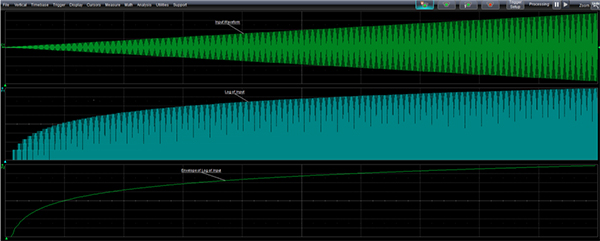Figure 1: The log amp compresses the input signal (top trace) by applying higher gain to the lowest amplitude signals and progressively lower gain to higher level signals. The middle trace shows the log of the input, while the bottom trace is the envelope of the log amp output. (Image source: Digi-Key Electronics)

The input signal (top trace) is an amplitude modulated carrier. The modulating signal is a linear ramp. The log amplifier output (middle trace) provides higher gain for the low-level signals and progressively lower gain as the signal level increases, producing a logarithmically weighted output signal. The bottom trace is the envelope of the log amp output that is an output option for a detector type log amp. A log amp applied before an ADC will compress the input signal to fit the fixed input range of the ADC.

Log amp topologies

There are two distinct log amp topologies: the multistage log amplifier and the DC log amplifier. The multistage log amplifier depends on sequential limiting in a series of amplifiers. This topology is most often used with high frequency signals up to several gigahertz and is typically found in radar and communications applications.

The DC log amplifier uses a diode or diode-connected transistor in the feedback loop of an operational amplifier (op amp). This type of log amp is limited to frequencies below 20 megahertz (MHz). Log amps using this technology are typically used with sensors in control applications.

The multistage log amp

With multistage log amps, a logarithmic amplitude response is achieved using a series of linear amplifiers with well-behaved overload limiting characteristics, the output of each driving the following stage as well as a summing circuit (Figure 2).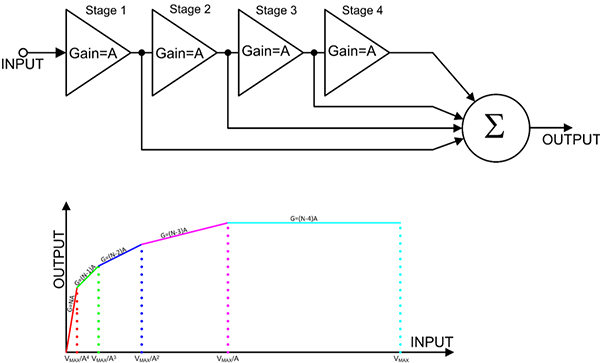Figure 2: A simple conceptual model of a series connection of multiple linear amplifiers whose individual outputs are summed is shown (top). The approach yields a logarithmic amplitude response as shown in the transfer function plot (bottom). (Image source: Digi-Key Electronics)

The amplifier string shown in Figure 2 uses four amplifiers, each with the same gain of A. Low amplitude signals—below the level to cause limiting in any of the stages—experience a gain of N × A, or 4 × A in this case. This is shown in the transfer function at the bottom of the Figure where the left-most (red) segment has a gain equal to N × A as indicated by the slope of the line segment for amplitudes between zero and VMAX / A4, where VMAX is the maximum input voltage.

As the input level is increased, at some point the last amplifier, Stage 4, will begin to limit. The overall gain will fall to (N - 1) × A, or 3 × A. The slope of the green segment, between input levels VMAX / A3 and VMAX / A4, represents this gain range. Similarly, as the input level continues to increase, the earlier stage amplifiers begin to limit successively. The dark blue segment’s gain is (N - 2) × A, the magenta segment has a gain of (N - 3) × A and the light blue segment’s gain is (N - 4) × A, or zero.

While this conceptual model is useful in explaining how a logarithmic response is developed using a series of amplifiers, it suffers from an unpleasant problem. There is an inherent propagation delay associated with each amplifier stage. Signal components from the first stage reach the summing circuit before those of later stages, distorting the output waveform. This can be corrected by altering the basic circuit (Figure 3).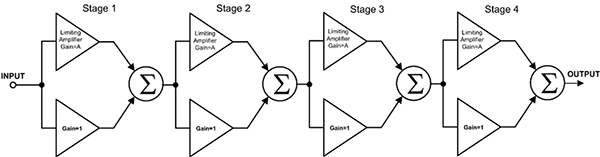Figure 3: The series log amplifier topology can be modified to eliminate delay by using a cascaded architecture with pairs of amplifiers. Each pair comprises a limiting amplifier to provide gain when necessary, and a unity gain buffer for when amplification is not required. Summation occurs at each stage eliminating delays. (Image source: Digi-Key Electronics)

This topology replaces the single stage amplifiers with pairs of amplifiers. Each pair comprises a limiting amplifier to supply gain when required, and a unity gain buffer if gain is not required. Summing occurs at each stage which eliminates the delay that occurs when using a single summer. For small signals, the limiting amplifiers provide the dominant path. As the signal amplitude increases, the last stage will begin to limit, permitting the unity gain amplifier of that stage to become the dominant input to the summer. Increasing the input level further causes earlier stages to limit in succession, resulting in an overall lowering of the gain.

A variant on the series amplifier topology is the successive detection log amp (Figure 4).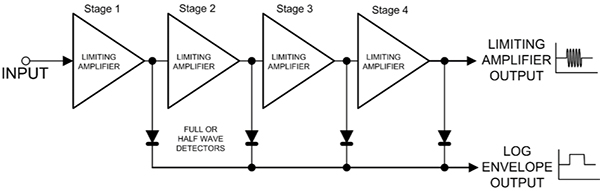Figure 4: The successive detection log amp adds peak detection after each stage. These outputs are then summed to create the amplitude envelope of the log amplified output signals. (Image source: Digi-Key Electronics)

The successive detection log amp uses the same limiting amplifier chain described previously but adds peak detection after each stage. These detector outputs are summed to create the amplitude envelope of the log amp output. Some versions also output the log amplified signals. Detectors can be implemented as half wave or full wave, depending on the circuit design. The log envelope is useful in applications that need to extract the detected signal level. Such applications include automatic gain controls and receiver signal strength indicators (RSSIs).

A good example of a commercial multistage demodulating log amp is Analog Devices’ AD8310ARMZ-REEL7 (Figure 5).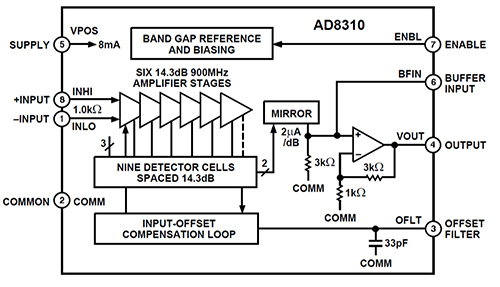Figure 5: The AD8310 multistage demodulating log amp cascades six amplifiers, each with a nominal gain of 14.3 dB (gain of 5.2) and a bandwidth of 900 MHz. (Image source: Analog Devices)

The AD8310 features a differential input that has a dynamic range of 95 dB over a bandwidth of 440 MHz, with ±0.4 dB log linearity. It cascades six amplifiers, each of which has a nominal gain of 14.3 dB (gain of 5.2) and a bandwidth of 900 MHz. Each amplifier drives a detector that has a current output which is converted to a voltage by an internal buffer amplifier and then output.

The DC log amplifier

As mentioned, an alternative log amp topology is the DC log amp. This uses a diode or diode-connected transistor in the feedback path of an op amp. The diode-connected transistor is the most commonly used configuration (Figure 6A). The voltage across the transistor base-emitter junction is proportional to the logarithm of the current through it. Having the diode-connected transistor in the feedback path of an op amp results in an output voltage that is proportional to the logarithm of the ratio of the input current to the emitter saturation (IES) current.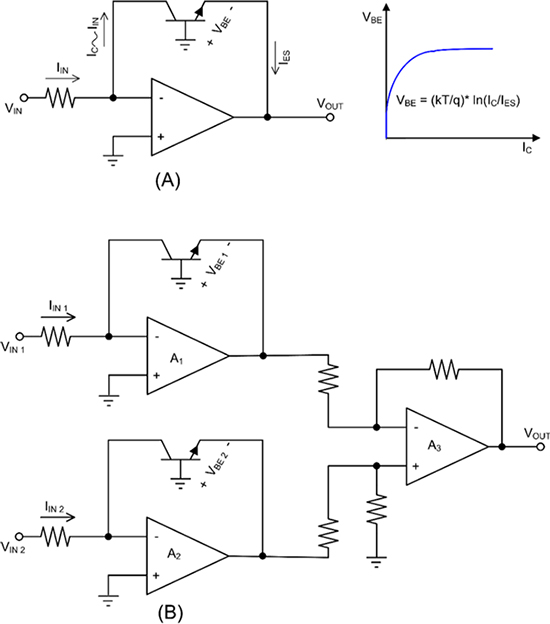Figure 6: A log amplifier can be realized by using a diode-connected transistor in the feedback path of an op amp (A). The temperature dependence of this type of log amp is greatly reduced by using two such amps connected differentially (B). (Image source: Digi-Key Electronics)

The simple configuration shown in Figure 6(A) has a limitation in that its output is temperature dependent as indicated in the equation, where T is the temp in degrees Kelvin, and by the saturation emitter current, IES. By configuring two such amplifiers as a differential pair as shown in Figure 6(B), this dependence can be greatly reduced. The differential version is a transimpedance amplifier that calculates the log of the ratio of IIN 2 / IIN 1 and has a voltage output. IIN 1 is generally set up as a fixed reference current.

The Texas Instruments LOG114AIRGVT is a DC log amp that has up to an eight decade dynamic range with a bandwidth of 5 MHz. It can be configured as a log amp or log ratio amp. In addition to the temperature compensated log amplifier, it includes two scaling op amps and a 2.5 volt voltage reference source (Figure 7).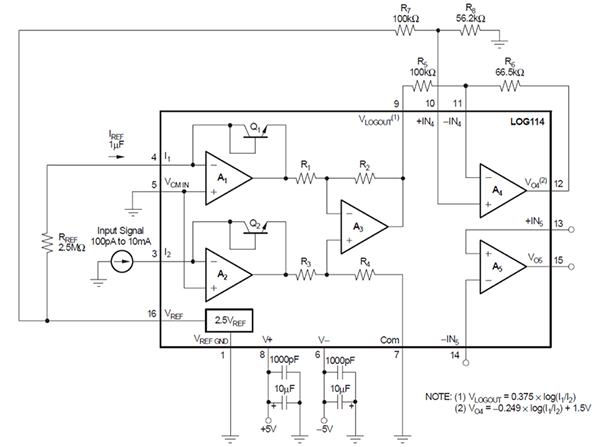Figure 7: The functional block diagram and associated external components of the LOG114 log amplifier. The amplifier is based on a temperature compensated circuit and includes two additional scaling amplifiers. (Image source: Texas Instruments)

Texas Instruments offers a circuit model for the LOG114 allowing designers to simulate their designs on the TINA-TI Texas Instruments circuit simulator (Figure 8).

Figure 8: The TINA-TI simulation of the LOG114 log amp model shows excellent log linearity over seven decades of input current. (Image source: Digi-Key Electronics)

This circuit uses the built-in 2.5 volt voltage reference to establish the reference current I1 at 1 microampere (µA). The accompanying transfer function shows a linear response over seven decades from 100 picoamperes (pA) to 1 milliampere (mA), a 140 dB current range. The log amp output is scaled using one of the two additional op amps to produce a transfer function equation: VOUT = -0.249 x log (I1 / I2) + 1.5 volts.

Conclusion

Log amplifiers offer the designer a technique to handle wide dynamic range signals whether baseband or RF. They do so by compressing a wide dynamic range signal into a fixed output range, preventing overflow and clipping conditions in the following stages. Log amp solutions are readily available and are frequently supported by online simulation tools to help in the design process.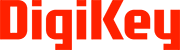Disclaimer: The opinions, beliefs, and viewpoints expressed by the various authors and/or forum participants on this website do not necessarily reflect the opinions, beliefs, and viewpoints of Digi-Key Electronics or official policies of Digi-Key Electronics.